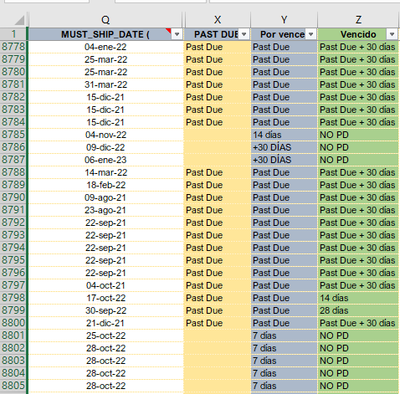cancel
Showing results for
Did you mean:

Fabric is Generally Available. Browse Fabric Presentations. Work towards your Fabric certification with the Cloud Skills Challenge.Regular Visitor

## ¿Como escribir esta fórmula?

Hola, solicitando de su apoyo...tengo esta formula en excel en la columna:

Por Vencer : =SI(Y(X8778="",Q8778<=Tabla!\$F\$1),"7 días",SI(Y(X8778="",Q8778<=Tabla!\$G\$1),"14 días",SI(Y(X8778="",Q8778<=Tabla!\$H\$1),"21 días",SI(Y(X8778="",Q8778<=Tabla!\$I\$1),"30 días",SI(X8778="Past Due","Past Due","+30 DÍAS")))))

Tengo esto datos de donde se toma la información para la formula:TablaHe tratado pero no logro replicarla en power bi

1 ACCEPTED SOLUTIONCommunity Support

HI @jalopez,

It seems like a common if statement expression to check conditions and return different segment flags.

Since power bi does not include row and column index, I think you need to add index fields to that table if your formula required to look up specific cell values.

Add an index column - Power Query | Microsoft Learn

BTW, please also convert these parameter fields to a table with different groups or indexes, then you can use the formula to look up them as conditions. (para table: group, date)

Sample calculate column formula:

``````Por Vencer =
IF (
AND (
Table[Past Due] = "",
Table[Ship Date] <= LOOKUPVALUE ( ParaTable[Date], ParaTable[Group], "F" )
),
"7 días",
IF (
AND (
Table[Past Due] = "",
Table[Ship Date] <= LOOKUPVALUE ( ParaTable[Date], ParaTable[Group], "G" )
),
"14 días",
IF (
AND (
Table[Past Due] = "",
Table[Ship Date] <= LOOKUPVALUE ( ParaTable[Date], ParaTable[Group], "H" )
),
"21 días",
IF (
AND (
Table[Past Due] = "",
Table[Ship Date] <= LOOKUPVALUE ( ParaTable[Date], ParaTable[Group], "I" )
),
"30 días",
IF ( Table[Past Due] = "Past Due", "Past Due", "+30 DÍAS" )
)
)
)
)``````

LOOKUPVALUE function (DAX) - DAX | Microsoft Learn

Regards,

Xiaoxin Sheng

Community Support Team _ Xiaoxin
If this post helps, please consider accept as solution to help other members find it more quickly.Community Support

HI @jalopez,

It seems like a common if statement expression to check conditions and return different segment flags.

Since power bi does not include row and column index, I think you need to add index fields to that table if your formula required to look up specific cell values.

Add an index column - Power Query | Microsoft Learn

BTW, please also convert these parameter fields to a table with different groups or indexes, then you can use the formula to look up them as conditions. (para table: group, date)

Sample calculate column formula:

``````Por Vencer =
IF (
AND (
Table[Past Due] = "",
Table[Ship Date] <= LOOKUPVALUE ( ParaTable[Date], ParaTable[Group], "F" )
),
"7 días",
IF (
AND (
Table[Past Due] = "",
Table[Ship Date] <= LOOKUPVALUE ( ParaTable[Date], ParaTable[Group], "G" )
),
"14 días",
IF (
AND (
Table[Past Due] = "",
Table[Ship Date] <= LOOKUPVALUE ( ParaTable[Date], ParaTable[Group], "H" )
),
"21 días",
IF (
AND (
Table[Past Due] = "",
Table[Ship Date] <= LOOKUPVALUE ( ParaTable[Date], ParaTable[Group], "I" )
),
"30 días",
IF ( Table[Past Due] = "Past Due", "Past Due", "+30 DÍAS" )
)
)
)
)``````

LOOKUPVALUE function (DAX) - DAX | Microsoft Learn

Regards,

Xiaoxin Sheng

Community Support Team _ Xiaoxin
If this post helps, please consider accept as solution to help other members find it more quickly.Announcements#### Power BI Monthly Update - November 2023

Check out the November 2023 Power BI update to learn about new features.#### Fabric Community News unified experience

Read the latest Fabric Community announcements, including updates on Power BI, Synapse, Data Factory and Data Activator.#### The largest Power BI and Fabric virtual conference

130+ sessions, 130+ speakers, Product managers, MVPs, and experts. All about Power BI and Fabric. Attend online or watch the recordings.Top Solution Authors
Top Kudoed Authors
Users online (3,687)